Next: Polynomials Up: Definitions Previous: Baseline   Contents

# Interferogram

The phase for a certain pixel in a single SLC image i is defined as: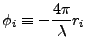(D14)

The complex interferogram minus reference phase is defined as: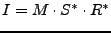(D15)

Where: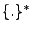denotes the complex conjugated;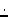denotes a pointwise multiplication;
I is the complex interferogram;
M is the complex master image;
S is the complex (resampled) slave image;
R is the complex (amplitude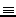1) reference phase;
The phase image (of complex interferogram minus reference phase) is defined as: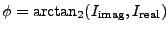(D16)

Where: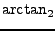is the four quadrant arc tangent;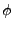is the phase image;
I is the complex interferogram;
Which is equal to (with an ambiguity of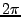)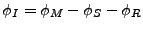(D17)

The reference phase is defined as (wheredenotes the range from the master satellite to a point on the reference surface)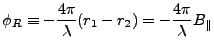(D18)

Which is the same as(D19)

Where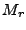denotes the phase of a point situated on the reference surface. (of course, in this definition the phase of the interferogram equals zero if there actually is no topography (and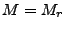).) The values of the (real valued) reference phase are stored in a 2d-polynomial of certain degree. The subtraction of the reference phaseis actually computed as: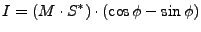(D20)

because the complex conjugated of the reference phaseequals: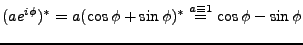(D21)

The complex coherence between two images is defined as (see ):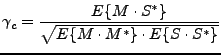(D22)

Where: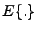is the expectation;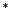is the complex conjugated;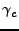is the complex coherence;
M is the complex master image;
S is the complex slave image (possibly minus (complex) reference phase: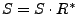;
The coherence is defined by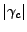, and its estimator as: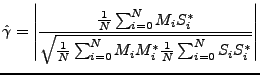(D23)

The correlation between two images is defined by (see ):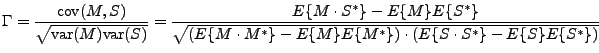(D24)

Thus the mean is first subtracted in comparison to the coherence. The coherence is equal to the correlation only if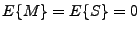.

A problem is that the estimator for the coherence and correlation is biased. For small window sizes its outcome is too high. This probably also causes the problems in the coarse coregistration, where the most likely offset is not selected based on its correlation value but on its consistency.Next: Polynomials Up: Definitions Previous: Baseline   Contents
Leijen 2009-04-14